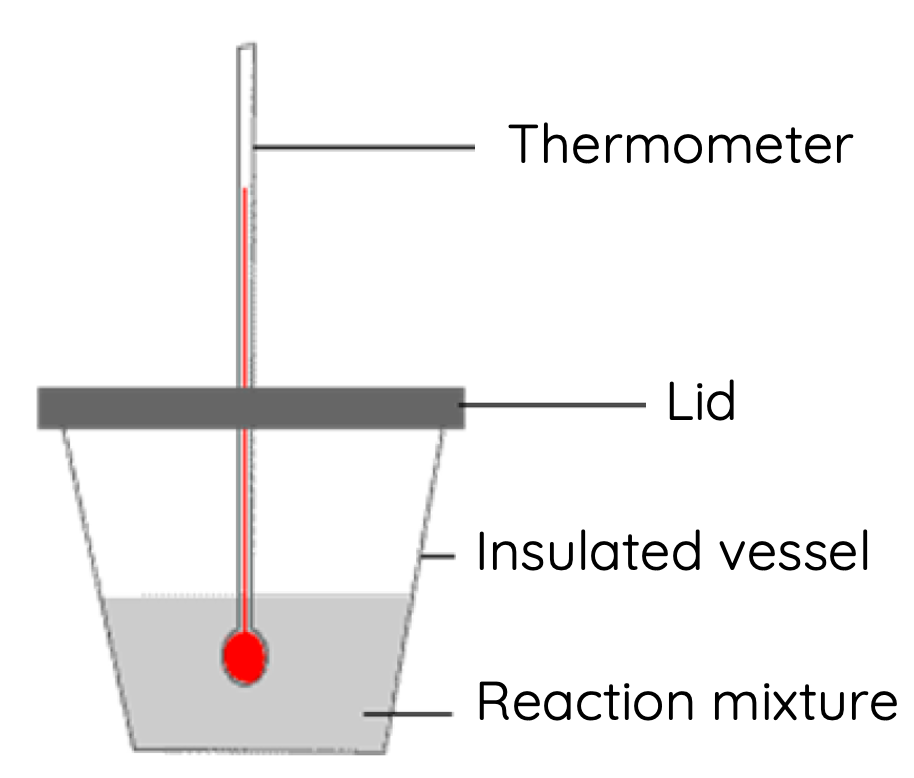# Enthalpy of Neutralisation Calorimetry Practical

This is part of the HSC Chemistry course under the topic Properties of Acids and Bases.

### HSC Chemistry Syllabus

• Conduct a practical investigation to measure the enthalpy of neutralisation (ACSCH093)

### Enthalpy of Neutralisation Calculation in Calorimetry

This video walks through how calorimetry can be used to estimate the molar enthalpy change of neutralisation.

### Measuring Enthalpy Change of Neutralisation Using Calorimetry

The enthalpy change of neutralisation is the amount of energy when 1 mole of water is formed from the reaction of an acid and a base.Since neutralisation reactions are exothermic, the final solution will increase in temperature.

By measuring the maximum change in temperature, the amount of energy produced by neutralisation and absorbed by the solution can be calculated:

$$q = mc \Delta T$$

Where:

• q = quantity of heat (amount of energy absorbed by the solution in J)
• m = mass of the final solution (unit depends on c - usually kg)
• c = the specific capacity of the final solution
• ΔT = the change in temperature given in Kelvin or degree Celsius

### What is Specific Heat Capacity?

The specific heat capacity is the amount of energy required to raise the temperature of a substance by 1 Kelvin (or ºC) per unit mass of that substance.

For example, pure water has a specific heat capacity of 4.18 x 103 J kg-1 K-1

This means 4.18 x 10J of energy is required to raise the temperature of 1 kg of water by 1 Kelvin or 1 degree Celsius.

### What is Calorimetry?

Calorimetry is the use of temperature change to determine the associated change in energy.

In neutralisation, the increase in temperature recorded is used to calculate the amount of energy produced by the exothermic reaction.

The amount of energy absorbed by the solution equals the amount of energy produced by neutralisation only when the following assumptions are made:

• No energy is lost to surrounding e.g. air
• Both acid and base solutions have equal initial temperatures

Sometimes, when the specific heat capacity of the solution is not known, the specific heat capacity of pure water can be used in the calculation. However, the validity and accuracy of this assumption depends on the concentration of the salt in the final solution.

### How to make calorimetry more accurate?

Some of amount of energy is bound to be lost to the surrounding environment, and not be absorbed by the solution. As a result, there will be some degree of inaccuracy when using calorimetry to determine the amount of energy produced by neutralisation.

The accuracy of calorimetry can be increased by minimising energy loss in the form of heat. This can be achieved by wrapping the beaker containing the solution with a layer of insulative material e.g. styrofoam and placing a lid over the beaker.

### Calculating Molar Enthalpy Change (∆H)

The enthalpy change of neutralisation is given by the equation:

$$\Delta H = -\frac{q}{n}$$

where:

• ΔH is the enthalpy change of neutralisation (exothermic)
• n is the number of moles of water formed
• q is the energy absorbed by the solution

There is a negative sign in the calculation of ∆because q represents the energy absorbed (+) by the final solution while ∆H represents the energy released (–) by neutralisation by mole of water formed.

Since the value of q will always be inaccurate due to unaccounted energy loss, there will be a discrepancy between the theoretical value of ∆H of neutralisation and the experimental value.